# Electronics and Communication Engineering - Materials and Components

### Exercise :: Materials and Components - Section 3

36.

Assertion (A): For most metals, Fermi energy EF is less than 10 eV.

Reason (R): Fermi level of a metal is given by EF = 3.64 x 10-19 (n)2/3 where n is number of free electrons/m3 of metal.

 A. Both A and R are true and R is correct explanation of A B. Both A and R are true but R is not correct explanation of A C. A is true but R is false D. A is false but R is true

Explanation:

No answer description available for this question. Let us discuss.

37.

The capacitance of an insulated conducting sphere of radius R in vacuum is

 A. 2p∈0R B. 4p∈0R C. 4p∈0R2 D. 4p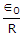Explanation:

No answer description available for this question. Let us discuss.

38.

The transition of electron from energy level W1 to W2 is associated with emission or absorption of electro magnetic radiation of frequency f such that

 A. hf = |W1 - W2| B. |W1 - W2|f = h C. hf = 0.5|W1 - W2| D. (hf)2 = |W1 - W2|

Explanation:

No answer description available for this question. Let us discuss.

39.

A material has N spins per m3. If Np are the dipoles per m3 parallel to field and Na are the dipoles per m3 anti-parallel to field, the magnetisation is

 A.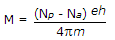B.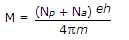C.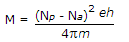D.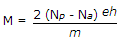Explanation:

No answer description available for this question. Let us discuss.

40.

Even at room temperature, an intrinsic semiconductor has some holes. These holes are due to

 A. electron B. doping C. thermal energy D. free electrons